• No products in the cart.

# Using TensorFlow Computer Vision to Read Sign Language

## Invent the Future with this Hands-on Step by Step Approach

### Import the Libraries

We start by importing the important libraries: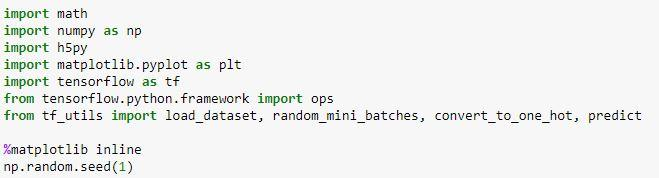?

Writing and running programs in TensorFlow has the following steps:

1. Create Tensors (variables) that are not yet executed/evaluated.

2. Write operations between those Tensors.

4. Create a Session.

5. Run the Session. This will run the operations you’d written above.

### One Hot encodings

One Hot encodings

Many times in deep learning you will have a y vector with numbers ranging from 0 to C-1, where C is the number of classes. If C is for example 4, then you might have the following y vector which you will need to convert as follows: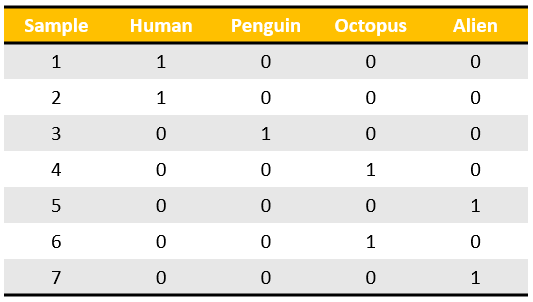?

This is called a “one hot” encoding, because in the converted representation exactly one element of each column is “hot” (meaning set to 1). To do this conversion in numpy, you might have to write a few lines of code. In Tensorflow, you can use one line of code:

• tf.one_hot(labels, depth, axis)

We will implement the function below to take one vector of labels and the total number of classes CC, and return the one hot encoding.

## Building the Network in TensorFlow with the Signs Dataset

Building the network in Tensorflow with the SIGNS Dataset

We will build an algorithm that would facilitate communications from a speech-impaired person to someone who doesn’t understand sign language.

• Training set: 1080 pictures (64 by 64 pixels) of signs representing numbers from 0 to 5 (180 pictures per number).
• Test set: 120 pictures (64 by 64 pixels) of signs representing numbers from 0 to 5 (20 pictures per number).

Note that this is a subset of the SIGNS dataset. The complete dataset contains many more signs.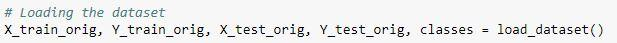?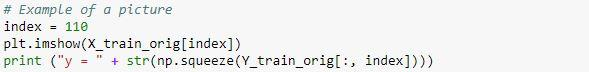?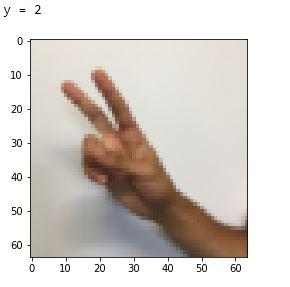?

As usual you flatten the image dataset, then normalize it by dividing by 255. On top of that, you will convert each label to a one-hot vector as shown in Figure 1. Run the cell below to do so.?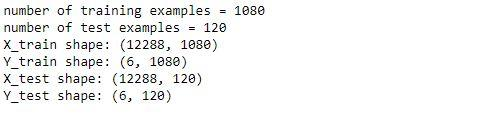?

Note that 12288 comes from 64×64×364×64×3. Each image is square, 64 by 64 pixels, and 3 is for the RGB colors. Please make sure all these shapes make sense to you before continuing.

The model is LINEAR -> RELU -> LINEAR -> RELU -> LINEAR -> SOFTMAX. The SIGMOID output layer has been converted to a SOFTMAX. A SOFTMAX layer generalizes SIGMOID to when there are more than two classes.

### Create Placeholders

Create placeholders

##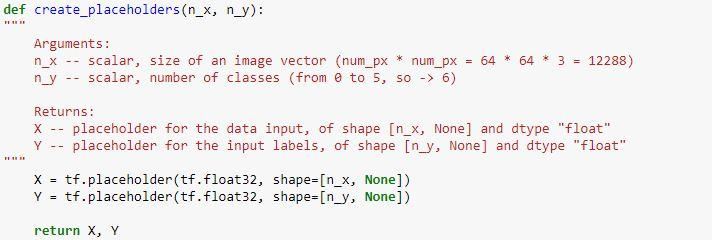?

### Initialize the Parameters

Initializing the parameters

##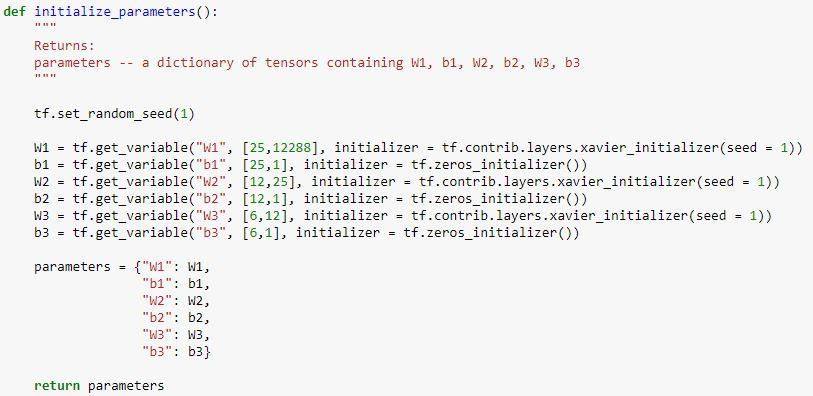?

### Forward Propagation

Forward propagation in Tensorflow

Computing cost

##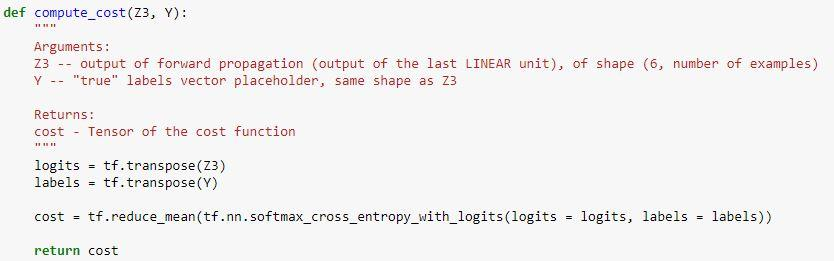?

Note When coding, we often use _ as a “throwaway” variable to store values that we won’t need to use later. Here, _ takes on the evaluated value of optimizer, which we don’t need (and c takes the value of the cost variable).

### Build and Test the Model

pi

Building the model

``def model(X_train, Y_train, X_test, Y_test, learning_rate = 0.0001, num_epochs = 1500, minibatch_size = 32, print_cost = True):``
``""”``
``Arguments:``
``X_train -- training set, of shape (input size = 12288, number of training examples = 1080)``
``Y_train -- test set, of shape (output size = 6, number of training examples = 1080)``
``X_test -- training set, of shape (input size = 12288, number of training examples = 120)``
``Y_test -- test set, of shape (output size = 6, number of test examples = 120)``
``learning_rate -- learning rate of the optimization``
``num_epochs -- number of epochs of the optimization loop``
``minibatch_size -- size of a minibatch``
``print_cost -- True to print the cost every 100 epochs``
``Returns:``
``parameters -- parameters learnt by the model. They can then be used to predict.``
``"""``
``ops.reset_default_graph()``
``tf.set_random_seed(1)``
``seed = 3``
``(n_x, m) = X_train.shape``
``n_y = Y_train.shape``
``costs = []``
`` ``
``X, Y = create_placeholders(n_x, n_y)``
`` ``
``# Initialize parameters``
``parameters = initialize_parameters()``
`` ``
``# Forward propagation``
``Z3 = forward_propagation(X, parameters)``
`` ``
``# Cost function``
``cost = compute_cost(Z3, Y)``
`` ``
``# Backpropagation using an AdamOptimizer.``
``optimizer = tf.train.AdamOptimizer(learning_rate=learning_rate).minimize(cost)``
`` ``
``init = tf.global_variables_initializer()``
``with tf.Session() as sess:``
``sess.run(init)``
`` ``
``# the training``
``for epoch in range(num_epochs):``
``epoch_cost = 0.``
``num_minibatches = int(m / minibatch_size)``
``seed = seed + 1``
``minibatches = random_mini_batches(X_train, Y_train, minibatch_size, seed)``
``for minibatch in minibatches:``
``(minibatch_X, minibatch_Y) = minibatch``
``_ , minibatch_cost = sess.run([optimizer, cost], feed_dict={X: minibatch_X, Y: minibatch_Y})``
`` ``
``epoch_cost += minibatch_cost / num_minibatches``
``# Printing the cost every epoch``
``if print_cost == True and epoch % 100 == 0:``
``print ("Cost after epoch %i: %f" % (epoch, epoch_cost))``
``if print_cost == True and epoch % 5 == 0:``
``costs.append(epoch_cost)``
``# plotting the cost``
``plt.plot(np.squeeze(costs))``
``plt.ylabel('cost')``
``plt.xlabel('iterations (per tens)')``
``plt.title("Learning rate =" + str(learning_rate))``
``plt.show()``
`` ``
``parameters = sess.run(parameters)``
``print ("Parameters have been trained!")``
`` ``
``correct_prediction = tf.equal(tf.argmax(Z3), tf.argmax(Y))``
`` ``
``# Calculate accuracy on the test set``
``accuracy = tf.reduce_mean(tf.cast(correct_prediction, "float"))``
``print ("Train Accuracy:", accuracy.eval({X: X_train, Y: Y_train}))``
``print ("Test Accuracy:", accuracy.eval({X: X_test, Y: Y_test}))``
``return parameters``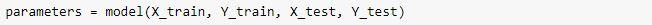?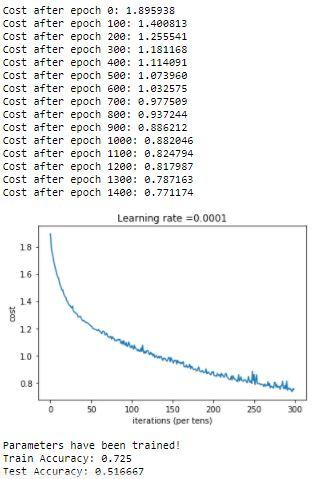?

August 9, 2021